Courses

# Determinant of a Matrix Mathematics Notes | EduRev

## Mathematics : Determinant of a Matrix Mathematics Notes | EduRev

The document Determinant of a Matrix Mathematics Notes | EduRev is a part of the Mathematics Course Algebra for IIT JAM Mathematics.
All you need of Mathematics at this link: Mathematics

The Determinant of a Matrix
To every square matrix A = [aij] of order n, we can associate a number (real or complex) called determinant of the square matrix A, where a = (i, j)th element of A. This may be thought of as a function which associates each square matrix with a unique number (real or complex).
If M is the set of square matrices, K is the set of numbers (real or complex) and f : M → K is defined by f (A) = k, where A ∈ M and k ∈ K, then f (A) is called the determinant of A. It is also denoted by | A | or det A or Δ.
If A=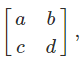then determinant of A is written as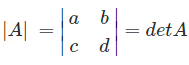For a 1×1 Matrix
Let A = [a] be the matrix of order 1, then determinant of A is defined to be equal to a.

For a 2×2 Matrix
For a 2×2 matrix (2 rows and 2 columns):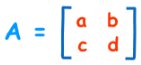The determinant is: |A| = ad − bc or the determinant of A equals a × d minus b × c. It is easy to remember when you think of a cross, where blue is positive that goes diagonally from left to right and red is negative that goes diagonally from right to left.Example: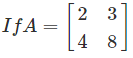|A| = 2 x 8 – 4 x 3
= 16 – 12
= 4

For a 3×3 Matrix
For a 3×3 matrix (3 rows and 3 columns):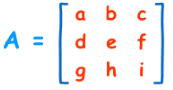The determinant is: |A| = a (ei − fh) − b (di − fg) + c (dh − eg). The determinant of A equals ‘a times e x i minus f x h minus b times d x i minus f x g plus c times d x h minus e x g’. It may look complicated, but if you carefully observe the pattern its really easy!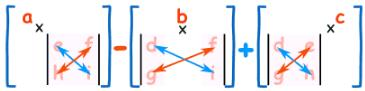To work out the determinant of a matrix 3×3:
• Multiply ‘a’ by the determinant of the 2×2 matrix that is not in a’s row or column.
• Likewise for ‘b’ and for ‘c’
• Sum them up, but remember the minus in front of the b
As a formula (remember the vertical bars || mean “determinant of”):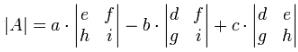The determinant of A equals ‘a’ times the determinant of e × i minus f × h minus ‘b’ times the determinant of d × i minus f × g plus ‘c’ times the determinant of d × h minus e × g.
Example: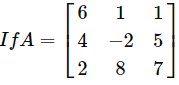|A|= 6×(−2×7 − 5×8) − 1×(4×7 − 5×2) + 1×(4×8 − (−2×2))
= 6×(−54) − 1×(18) + 1×(36)
= −306

For 4×4 Matrices and Higher
The pattern continues for the determinant of a matrix 4×4:
• plus a times the determinant of the matrix that is not in a’s row or column,
minus b times the determinant of the matrix that is not in b’s row or column,
plus c times the determinant of the matrix that is not in c’s row or column,
minus d times the determinant of the matrix that is not in d’s row or column,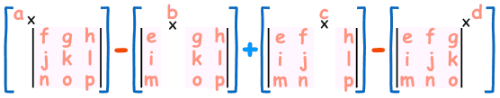As a formula: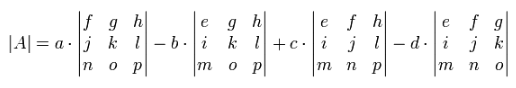Notice the +−+− pattern (+a… −b… +c… −d…).

Solved Examples For You
Question 1: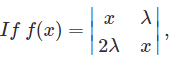then f(λx) – f(x) is equal to:
A. x (λ2 – 1)
B. 2λ (x2 – 1)
C. λ2(x2 – 1)
D. x2 (λ2 – 1)
Solution: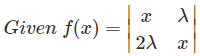⇒ f(x) = x2 − 2λ2
Therefore, f(λx) − f(x) = (λx)2 − 2λ2 − (x2−2λ2)
2x2−2λ2−x2+2λ2
=x22−1)
So, option D is correct.

Question 2: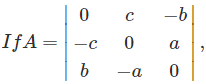then (a2 + b2 – c2) |A| =
1. abc
2. a + b + c
3. a3 + b3 + c3
4. 0
Solution: |A| = 0 × (a2) −c(− ab) − b(ac) = 0 + abc – abc = 0

Minor of a Determinant
A minor is defined as a value computed from the determinant of a square matrix which is obtained after crossing out a row and a column corresponding to the element that is under consideration. Minor of an element aij of a determinant is the determinant obtained by deleting its ith row and jth column in which element aij lies. Minor of an element aij is denoted by Mij.

Cofactor of a Determinant
The cofactor is defined as the signed minor. Cofactor of an element aij, denoted by Aij is defined by A = (–1)i+j M, where M is minor of aij.
Note
We note that if the sum i+j is even, then Aij = Mij, and that if the sum is odd, then Aij = −Mij.
Hence, the only difference between the related minor entries and cofactors may be a sign change or nothing at all.
Whether  or Aij = Mij or Aij = −Mij
has a pattern for square matrices as illustrated: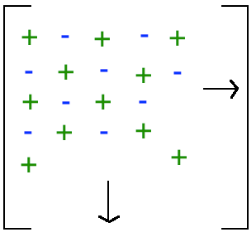For example C12 = −M12. Of course, if you forget, you can always use the formula Cij = (−1)i+j Mij,
Here, C12=(−1)1+2 Mij = (−1)3 Mij = −Mij

Example: Find the minors and cofactors of all the elements of the determinant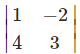Solution: Minor of the element aij is Mij.
Here a11 = 1. So M11 = Minor of a11 = 3
M12 = Minor of the element a12 = 4
M21 = Minor of the element a21 = –2
M22 = Minor of the element a22 = 1
Now, cofactor of aij is Aij. So,
A11 = (–1)1+1, M11 = (–1)2 (3) = 3
A12 = (–1)1+2, M12 = (–1)3 (4) = –4
A21 = (–1)2+1, M21 = (–1)3 (–2) = 2
A22 = (–1)2+2, M22 = (–1)4 (1) = 1

Solved Examples for You
Question 1: Let A=[aij]n×n be a square matirx and let cij be cofactor of aij in A. If C=[cij], then
1. |A| = |C|
2.|C| = |A|n-1
3. |C| = |A|n-2
4. none of these
Solution: We know that adjA = CT where C is the cofactor matrix of A.
=|A|n-1 where n it the order of the square matrix.

Question 2: The minors and cofactors of -4 and 9 in determinant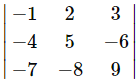are respectively
A. 42, 42; 3, 3
B. 42, -42; 3, 3
C. 42, -42; 3, -3
D. 42, 3; 42, 3
Solution: Minor of -4 is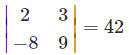Cofactor of -4 is (−1)1+2(42) = −42
Minor of 9 is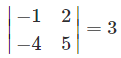Cofactor of 9 is (−1)3+3 .(3) = 3. Therefore, the answer is option B

Offer running on EduRev: Apply code STAYHOME200 to get INR 200 off on our premium plan EduRev Infinity!

58 docs

,

,

,

,

,

,

,

,

,

,

,

,

,

,

,

,

,

,

,

,

,

;